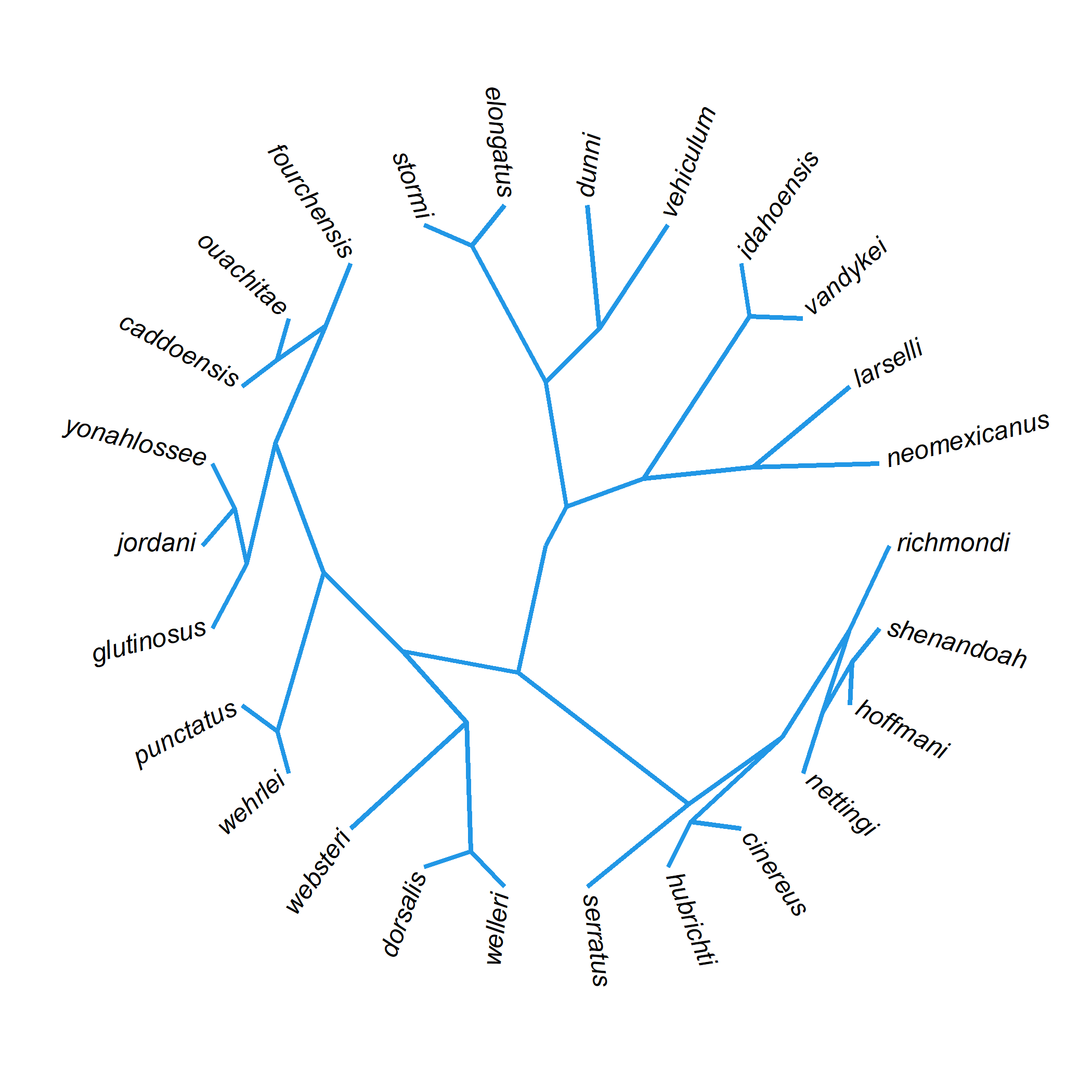## Monday, January 2, 2023

### On a strange algorithm for plotting trees in R

Here’s a weird way to plot a phylogenetic tree in R that that I haven’t found a use for yet (but still might).

First, get the coordinates of the nodes of the tree we’re going to plot.

Then, iterating over all the tips of the tree, compute the set of ancestors from the tip to the global root. For this, we’ll use the phangorn package, but we could’ve also done it via a post-order traversal.

Finally, graph a line (with `type="s"` for a square phylogram) from through the coordinates on each path from the root to each tip.

This is what this looks like in R.

``````## load libraries
library(phytools)
library(phangorn)
data(mammal.tree)
## compute coordinates of tree to plot
plotTree(mammal.tree,color="transparent",fsize=0.7,
ftype="i")
## get coordinates from "last_plot.phylo"
obj<-get("last_plot.phylo",envir=.PlotPhyloEnv)
for(i in 1:Ntip(mammal.tree)){
## get the ancestors of every tip
aa<-c(i,Ancestors(mammal.tree,i))
## plot the line segments, using type="s", from
## the root
lines(obj\$xx[aa],obj\$yy[aa],type="s",lwd=5,
col=palette())
}
``````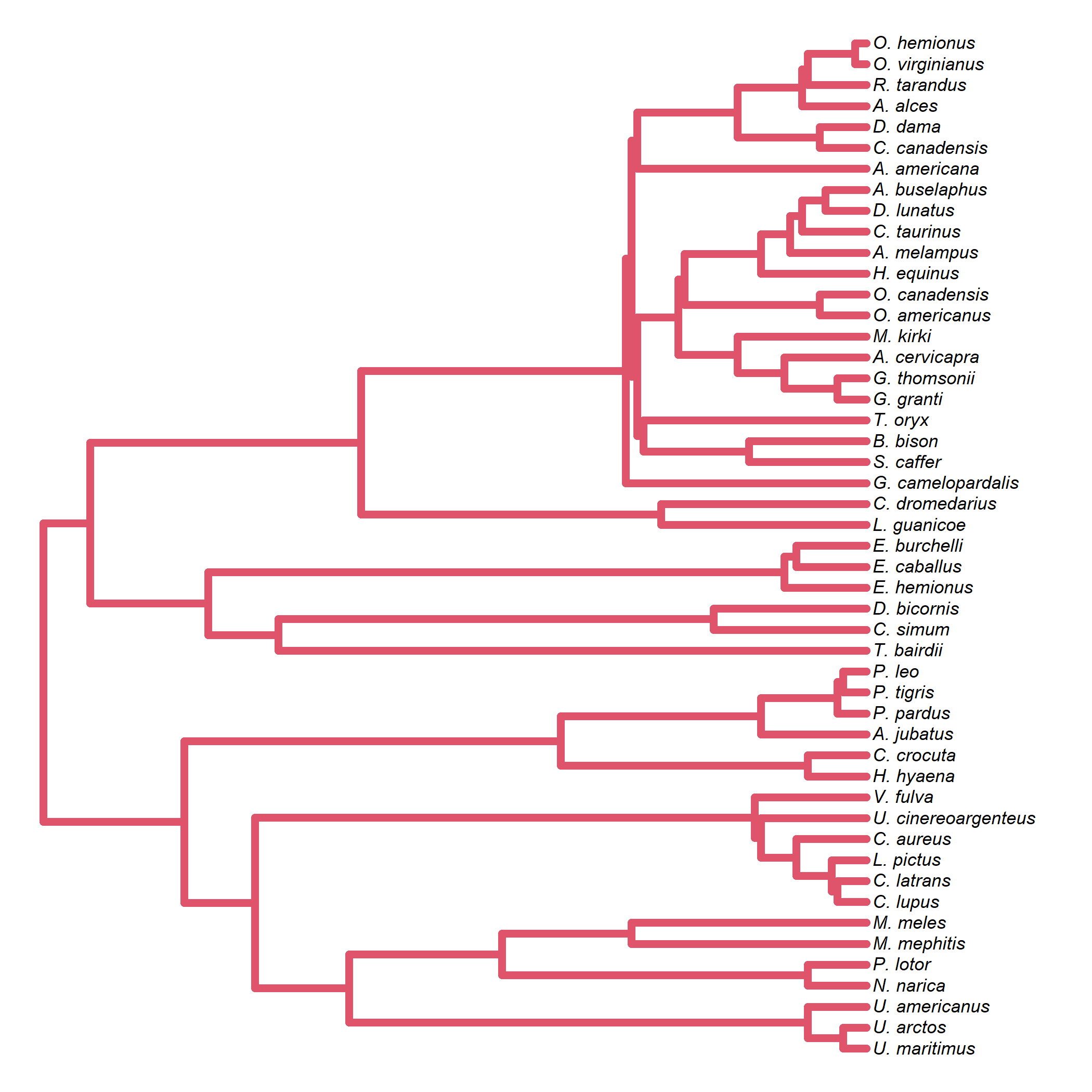To see that the tree has been graphed this way, let’s assign an α transparency value to our color and see that edges near the root are plotted many times, while edges near the tips are plotted few times or only once.

``````plotTree(mammal.tree,color="transparent",fsize=0.7,
ftype="i")
obj<-get("last_plot.phylo",envir=.PlotPhyloEnv)
for(i in 1:Ntip(mammal.tree)){
aa<-c(i,Ancestors(mammal.tree,i))
lines(obj\$xx[aa],obj\$yy[aa],type="s",lwd=5,
col=make.transparent(palette(),0.1))
}
``````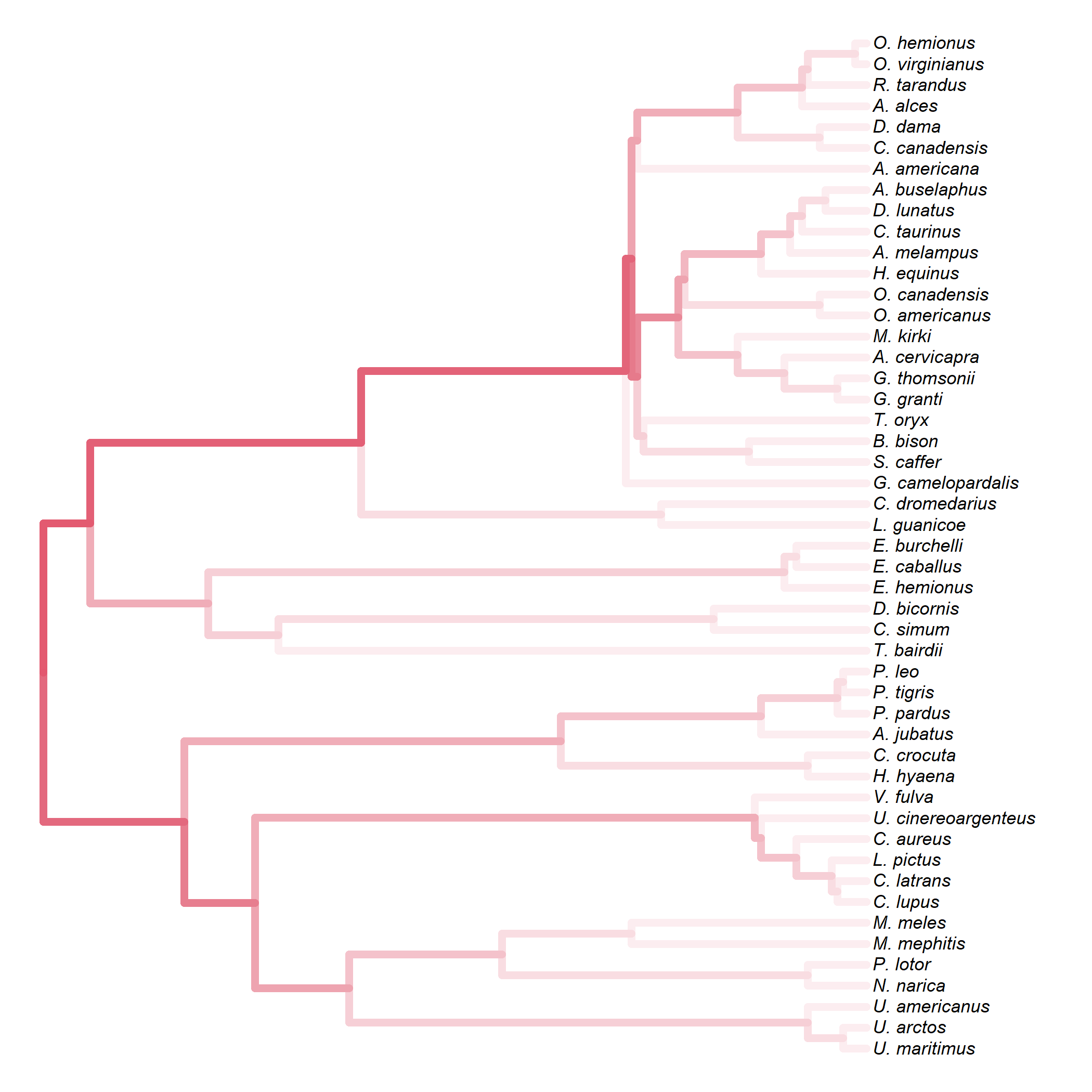The only obvious advantage of using this algorithm, compared to plotting the edges and vertical connecting lines, one by one is that it allows us to more easily use “beveled” line join style. (This has no meaning if each line segment is plotted separately.)

For example:

``````par(ljoin=2,lend=1)
plotTree(mammal.tree,color="transparent",fsize=0.7,
ftype="i")
obj<-get("last_plot.phylo",envir=.PlotPhyloEnv)
for(i in 1:Ntip(mammal.tree)){
aa<-c(i,Ancestors(mammal.tree,i))
lines(obj\$xx[aa],obj\$yy[aa],type="s",lwd=7,
col=palette())
}
``````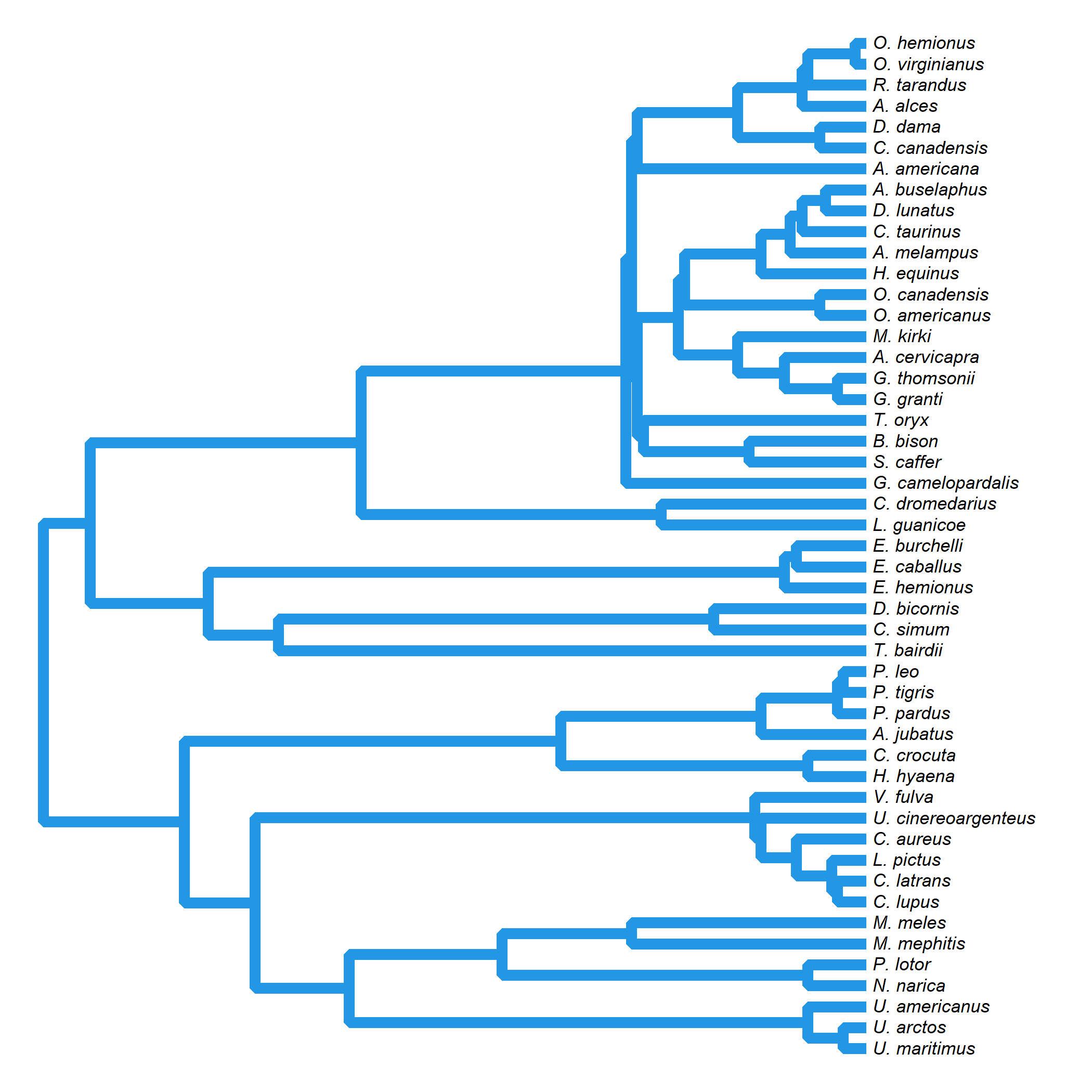This compared to with `phytools::plotTree`.

``````par(ljoin=2,lend=1)
plotTree(mammal.tree,fsize=0.7,ftype="i",lwd=7,
color=palette())
``````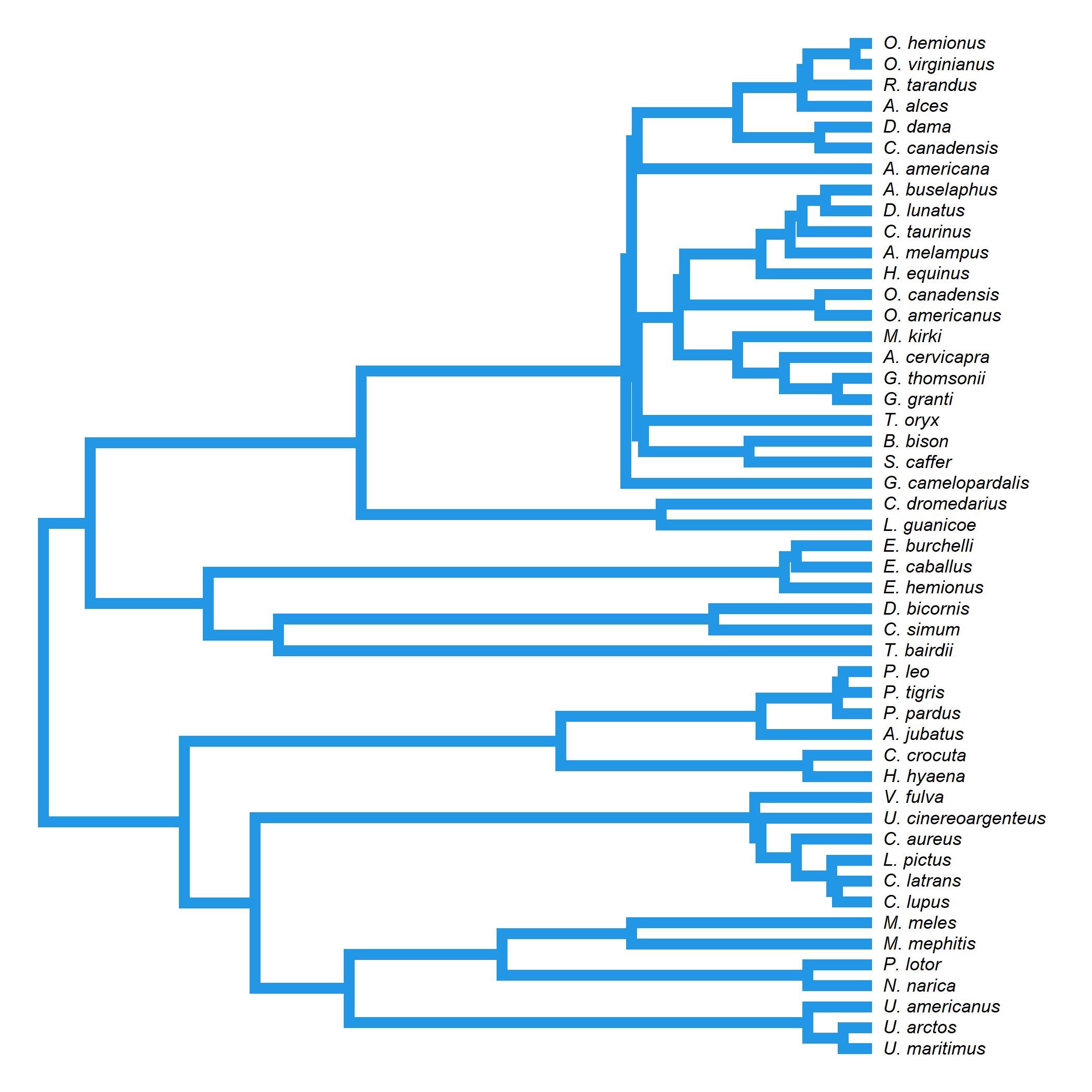Lastly, just to see what accidental creation results, let’s try `type="fan"`.

``````data(salamanders)
par(ljoin=2,lend=1)
plotTree(salamanders,color="transparent",
ftype="i",type="fan")
obj<-get("last_plot.phylo",envir=.PlotPhyloEnv)
for(i in 1:Ntip(salamanders)){
aa<-c(i,Ancestors(salamanders,i))
lines(obj\$xx[aa],obj\$yy[aa],type="s",lwd=3,
col=palette())
}
``````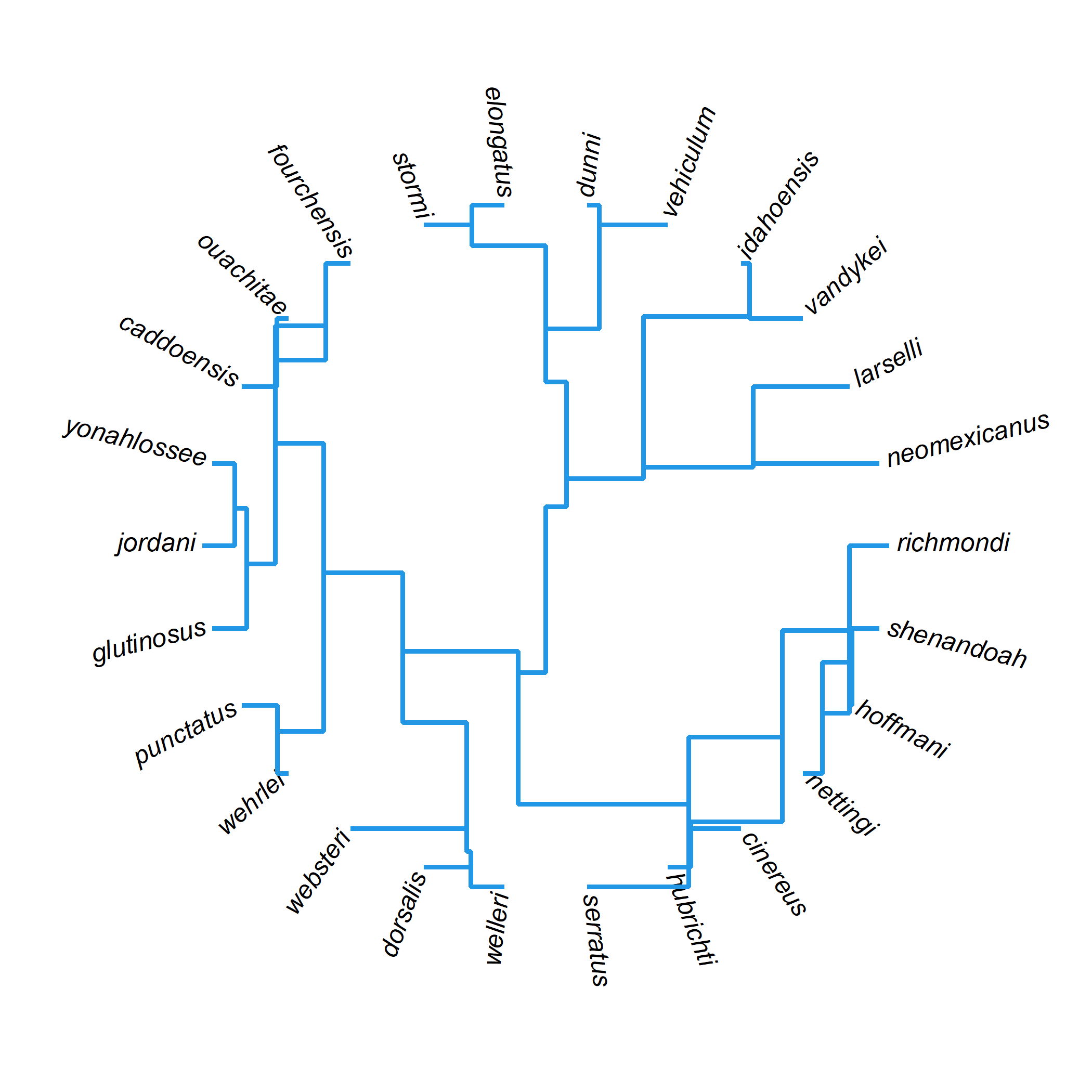Yikes.

Maybe a “radial” style will come out better.

``````par(ljoin=2,lend=1)
plotTree(salamanders,color="transparent",
ftype="i",type="fan")
obj<-get("last_plot.phylo",envir=.PlotPhyloEnv)
for(i in 1:Ntip(salamanders)){
aa<-c(i,Ancestors(salamanders,i))
lines(obj\$xx[aa],obj\$yy[aa],lwd=3,
col=palette())
}
``````